# Equivalent Ratios

This worksheet has rows of equivalent ratios, each with either the first term or second term left blank. One ratio in each row will be written with both terms. The student will fill in the missing terms.

## Shuffle

 List the ratios in order from smallest terms to largest terms.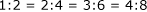List the ratios in any order.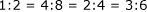## Gaps

 Do not allow gaps in the list of ratios.Allow gaps.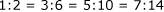## Show Simplest Ratio

 Always show the simplest ratio complete.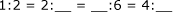Show any ratio in the list complete.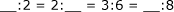## Multiple worksheets

Create different worksheets using these selections.